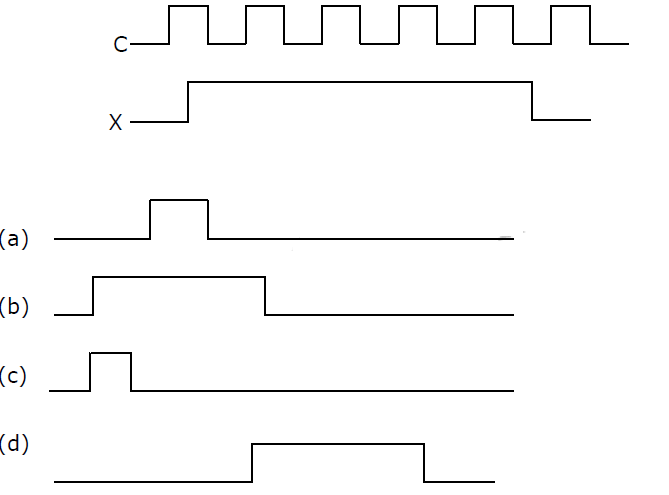# GATE | GATE-CS-2001 | Question 33

Consider the following circuit with initial state Q0 = Q1 = 0. The D Flip-flops are positive edged triggered and have set up times 20 nanosecond and hold times 0.Consider the following timing diagrams of X and C; the clock period of C <= 40 nanosecond. Which one is the correct plot of Y?(A) a
(B) b
(C) c
(D) d

Explanation: Set up time and hold times are given just to ensure that edge triggering works properly.

1. Since clock is positive edge triggered, so first positive edge trigger: Since X is 0, so output will be 0. Also, Q0 and Q0′ are 0 and 1 respectively.
2. Now, in second step, Q0′ would be 1 because of setup time of flip-flop is 20 ns and clock-period is ≥ 40 ns.
Therefore, second positive edge trigger: Because of X is 1 and Q0′ is 1, so output is 1.
3. Now, Q0′ will became 0, but output Y won’t change as the flip-flop is positive edge triggered.
Third positive edge trigger: Because of X is 1 and Q0′ is 0, so output is 0.
4. Now, output never changes back to 1 as Q0′ is always 0 and when Q0′ finally becomes 1, X is 0.

So, option (A) is correct.

Please comment below if you find anything wrong in the above post.

Quiz of this Question

My Personal Notes arrow_drop_up
Article Tags :

1

Please write to us at contribute@geeksforgeeks.org to report any issue with the above content.